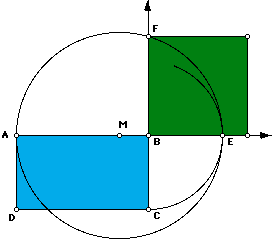# Squaring A RectangleTo squaring a rectangle means to construct a square with an area equal to the area of a given rectangle. You may square a rectangle using only a compass and a straight edge in the following activity. (The proof may be found in [1, p.51].)2. Extend side AB.
3. Draw an arc centered at B with radius BC. Let E be the intersection of the arc and ray AB.
4. Construct the midpoint M of segment AE.
5. Construct a circle centered at M with radius OA.
6. Extend side CB.
7. Let F be the point where the circle and ray CB intersect.
8. Then BF is the side of the desired square. Complete by constructing a square with side BF.

This has been done on Geometer's Sketchpad. To see this relationship between a square and a rectangle click on squaring a rectangle sketch.

If you have your own rectangle that you wish to square, you may use the squaring a rectangle script.http://www.geom.umn.edu/~huberty/math5337/groupe/squarerect.html